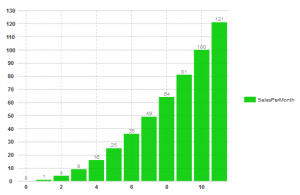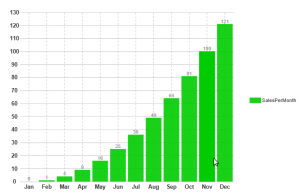﻿ Using ValueLabels in Wijmo Charts# Using ValueLabels in Wijmo Charts

The annotation along each axis is an important part of any chart. Wijmo Chart annotates the axes with numbers/datetime values based on Series data. You may format the values using AnnoFormatString property but sometimes we have a requirement to display strings along an axis instead of numeric values (for example, you may be charting product prices and want to display the product names along the X-axis). In such cases, we may use ValueLabels Annotation which displays text defined at a specific axis coordinate. This annotation is useful in labeling only specific coordinates or when producing annotation in a form that the chart does not provide. ValueLabels annotation can be used for any axis and with any chart type with the exception of Pie charts. In this blog, we will see how we can implement the same in Wijmo charts. Suppose we have a Bar chart with some unbound data as below:

``````  double[] unitsSold = new double;
double[] month = new double;

//populate the array
int i = 0;
for (i = 0; i < 12; i++)
{
unitsSold[ i ] = i;
month[ i ] = i * i;
}

//create an object of chart series and add data
BarChartSeries bcs = new BarChartSeries();
bcs.Label = "SalesPerMonth";

``````

If we run the above code, then we will get a chart like below:However, we can enhance this chart by displaying the respective month names instead of number with the help of ValueLabels. For this, we need to create objects of ValueLabel depending on our requirement and then add them in the ValueLabelList collection. Remember,Value labels are only displayed if the axis AnnoMethod property is set to ValueLabels. The code for adding Valuelabels will look like:

``````
//array consisting names of the months
string[] monthNames = DateTimeFormatInfo.CurrentInfo.MonthNames;

for (i = 0; i < monthNames.Length - 1; i++)
{
//create object of ValueLabel class
ValueLabel vl = new ValueLabel();
// set the x-axis value which you want to replace
vl.NumericValue = i;
// set the desired value which you want to show
vl.Text = monthNames[i].Substring(0, 3);
// add it to the ValueLabel collection
}

//set the annotation to valuelabels instead of values.
C1BarChart1.Axis.X.AnnoMethod = ChartAxisAnnoMethod.ValueLabels;

``````

The final chart with ValueLabels will displayed as :ValueLabels can also be modified at design time through the ValueLabel Collection Editor. Download Sample (C#) Download Sample (VB)GrapeCity

GrapeCity Developer Tools